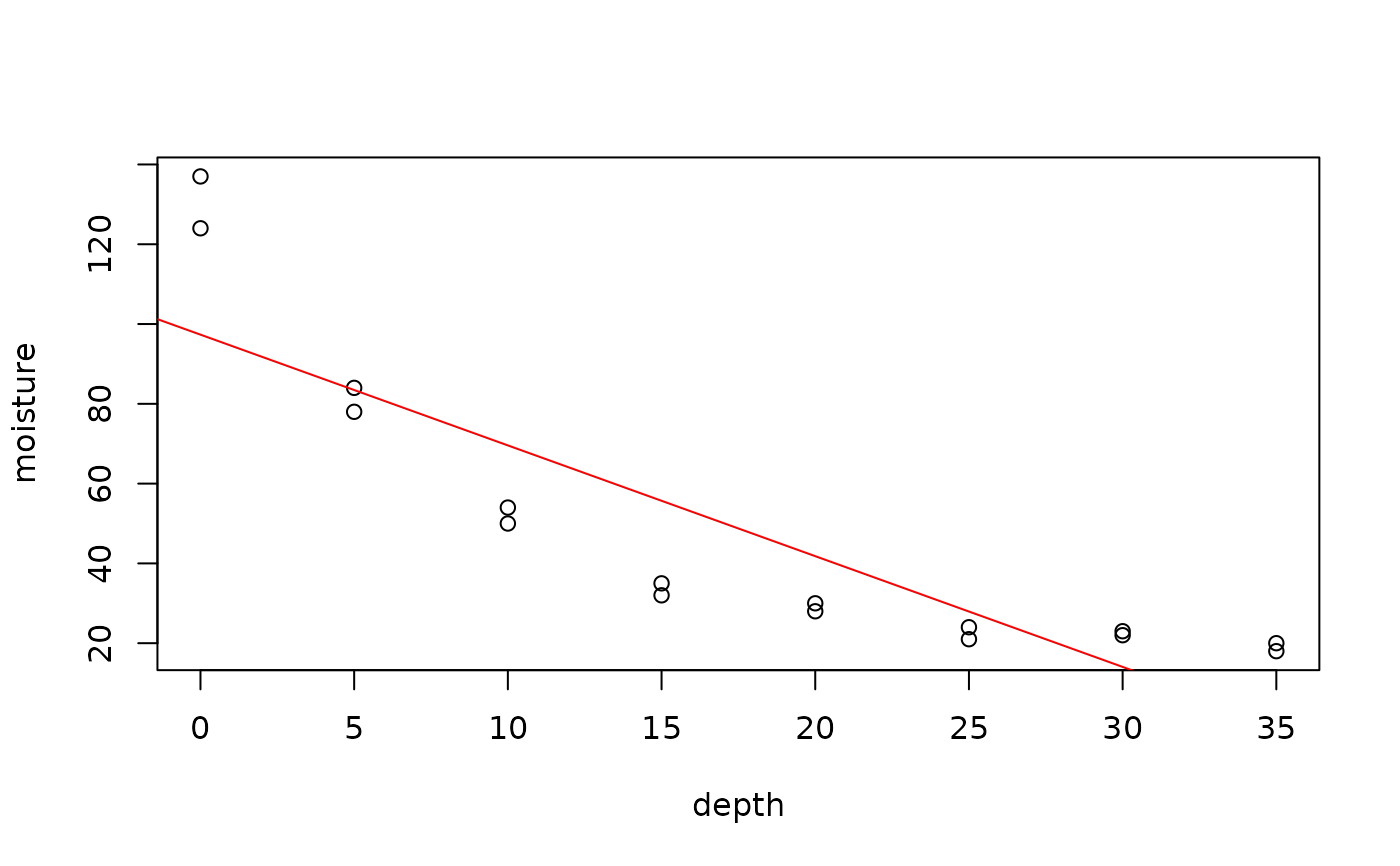Data for Exercise 9.32

Moisture

## Format

A data frame/tibble with 16 observations on four variables

depth

a numeric vector

moisture

g of water per 100 g of dried sediment

lnmoist

a numeric vector

depthsq

a numeric vector

## Source

Davis, J. C. (1986), Statistics and Data Analysis in Geology, 2d. ed., John Wiley and Sons, New York, pp. 177, 185.

## Examples


plot(moisture ~ depth, data = Moisture)
model <- lm(moisture ~ depth, data = Moisture)
abline(model, col = "red")plot(resid(model) ~ depth, data = Moisture)rm(model)